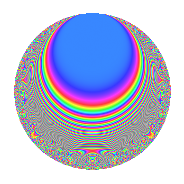# Properties

 Label 802.2.aLevel 802 Weight 2 Character orbit a Rep. character $$\chi_{802}(1,\cdot)$$ Character field $$\Q$$ Dimension 33 Newforms 6 Sturm bound 201 Trace bound 3

# Related objects

## Defining parameters

 Level: $$N$$ = $$802 = 2 \cdot 401$$ Weight: $$k$$ = $$2$$ Character orbit: $$[\chi]$$ = 802.a (trivial) Character field: $$\Q$$ Newforms: $$6$$ Sturm bound: $$201$$ Trace bound: $$3$$ Distinguishing $$T_p$$: $$3$$

## Dimensions

The following table gives the dimensions of various subspaces of $$M_{2}(\Gamma_0(802))$$.

Total New Old
Modular forms 102 33 69
Cusp forms 99 33 66
Eisenstein series 3 0 3

The following table gives the dimensions of the cuspidal new subspaces with specified eigenvalues for the Atkin-Lehner operators and the Fricke involution.

$$2$$$$401$$FrickeDim.
$$+$$$$+$$$$+$$$$9$$
$$+$$$$-$$$$-$$$$7$$
$$-$$$$+$$$$-$$$$12$$
$$-$$$$-$$$$+$$$$5$$
Plus space$$+$$$$14$$
Minus space$$-$$$$19$$

## Trace form

 $$33q$$ $$\mathstrut +\mathstrut q^{2}$$ $$\mathstrut +\mathstrut 2q^{3}$$ $$\mathstrut +\mathstrut 33q^{4}$$ $$\mathstrut +\mathstrut 2q^{5}$$ $$\mathstrut +\mathstrut 2q^{6}$$ $$\mathstrut -\mathstrut 8q^{7}$$ $$\mathstrut +\mathstrut q^{8}$$ $$\mathstrut +\mathstrut 41q^{9}$$ $$\mathstrut +\mathstrut O(q^{10})$$ $$33q$$ $$\mathstrut +\mathstrut q^{2}$$ $$\mathstrut +\mathstrut 2q^{3}$$ $$\mathstrut +\mathstrut 33q^{4}$$ $$\mathstrut +\mathstrut 2q^{5}$$ $$\mathstrut +\mathstrut 2q^{6}$$ $$\mathstrut -\mathstrut 8q^{7}$$ $$\mathstrut +\mathstrut q^{8}$$ $$\mathstrut +\mathstrut 41q^{9}$$ $$\mathstrut +\mathstrut 2q^{10}$$ $$\mathstrut +\mathstrut 8q^{11}$$ $$\mathstrut +\mathstrut 2q^{12}$$ $$\mathstrut +\mathstrut 4q^{13}$$ $$\mathstrut +\mathstrut 4q^{14}$$ $$\mathstrut +\mathstrut 8q^{15}$$ $$\mathstrut +\mathstrut 33q^{16}$$ $$\mathstrut -\mathstrut 10q^{17}$$ $$\mathstrut +\mathstrut 5q^{18}$$ $$\mathstrut -\mathstrut 2q^{19}$$ $$\mathstrut +\mathstrut 2q^{20}$$ $$\mathstrut -\mathstrut 4q^{21}$$ $$\mathstrut -\mathstrut 4q^{22}$$ $$\mathstrut +\mathstrut 8q^{23}$$ $$\mathstrut +\mathstrut 2q^{24}$$ $$\mathstrut +\mathstrut 31q^{25}$$ $$\mathstrut -\mathstrut 4q^{27}$$ $$\mathstrut -\mathstrut 8q^{28}$$ $$\mathstrut -\mathstrut 6q^{29}$$ $$\mathstrut -\mathstrut 12q^{31}$$ $$\mathstrut +\mathstrut q^{32}$$ $$\mathstrut -\mathstrut 8q^{33}$$ $$\mathstrut -\mathstrut 2q^{34}$$ $$\mathstrut -\mathstrut 40q^{35}$$ $$\mathstrut +\mathstrut 41q^{36}$$ $$\mathstrut -\mathstrut 20q^{37}$$ $$\mathstrut -\mathstrut 2q^{38}$$ $$\mathstrut -\mathstrut 32q^{39}$$ $$\mathstrut +\mathstrut 2q^{40}$$ $$\mathstrut -\mathstrut 2q^{41}$$ $$\mathstrut -\mathstrut 16q^{42}$$ $$\mathstrut -\mathstrut 28q^{43}$$ $$\mathstrut +\mathstrut 8q^{44}$$ $$\mathstrut +\mathstrut 10q^{45}$$ $$\mathstrut -\mathstrut 8q^{46}$$ $$\mathstrut -\mathstrut 16q^{47}$$ $$\mathstrut +\mathstrut 2q^{48}$$ $$\mathstrut +\mathstrut 13q^{49}$$ $$\mathstrut +\mathstrut 15q^{50}$$ $$\mathstrut +\mathstrut 28q^{51}$$ $$\mathstrut +\mathstrut 4q^{52}$$ $$\mathstrut -\mathstrut 20q^{53}$$ $$\mathstrut +\mathstrut 8q^{54}$$ $$\mathstrut -\mathstrut 28q^{55}$$ $$\mathstrut +\mathstrut 4q^{56}$$ $$\mathstrut -\mathstrut 12q^{57}$$ $$\mathstrut -\mathstrut 2q^{58}$$ $$\mathstrut -\mathstrut 22q^{59}$$ $$\mathstrut +\mathstrut 8q^{60}$$ $$\mathstrut -\mathstrut 8q^{61}$$ $$\mathstrut -\mathstrut 28q^{62}$$ $$\mathstrut -\mathstrut 68q^{63}$$ $$\mathstrut +\mathstrut 33q^{64}$$ $$\mathstrut -\mathstrut 12q^{65}$$ $$\mathstrut -\mathstrut 8q^{66}$$ $$\mathstrut -\mathstrut 34q^{67}$$ $$\mathstrut -\mathstrut 10q^{68}$$ $$\mathstrut -\mathstrut 32q^{69}$$ $$\mathstrut -\mathstrut 16q^{70}$$ $$\mathstrut -\mathstrut 8q^{71}$$ $$\mathstrut +\mathstrut 5q^{72}$$ $$\mathstrut -\mathstrut 18q^{73}$$ $$\mathstrut -\mathstrut 14q^{75}$$ $$\mathstrut -\mathstrut 2q^{76}$$ $$\mathstrut -\mathstrut 8q^{77}$$ $$\mathstrut +\mathstrut 16q^{79}$$ $$\mathstrut +\mathstrut 2q^{80}$$ $$\mathstrut +\mathstrut 97q^{81}$$ $$\mathstrut +\mathstrut 10q^{82}$$ $$\mathstrut -\mathstrut 20q^{83}$$ $$\mathstrut -\mathstrut 4q^{84}$$ $$\mathstrut +\mathstrut 20q^{86}$$ $$\mathstrut -\mathstrut 4q^{87}$$ $$\mathstrut -\mathstrut 4q^{88}$$ $$\mathstrut +\mathstrut 2q^{89}$$ $$\mathstrut +\mathstrut 14q^{90}$$ $$\mathstrut -\mathstrut 40q^{91}$$ $$\mathstrut +\mathstrut 8q^{92}$$ $$\mathstrut +\mathstrut 4q^{93}$$ $$\mathstrut +\mathstrut 28q^{94}$$ $$\mathstrut -\mathstrut 12q^{95}$$ $$\mathstrut +\mathstrut 2q^{96}$$ $$\mathstrut -\mathstrut 6q^{97}$$ $$\mathstrut +\mathstrut 17q^{98}$$ $$\mathstrut +\mathstrut O(q^{100})$$

## Decomposition of $$S_{2}^{\mathrm{new}}(\Gamma_0(802))$$ into irreducible Hecke orbits

Label Dim. $$A$$ Field CM Traces A-L signs $q$-expansion
$$a_2$$ $$a_3$$ $$a_5$$ $$a_7$$ 2 401
802.2.a.a $$1$$ $$6.404$$ $$\Q$$ None $$1$$ $$-2$$ $$-2$$ $$0$$ $$-$$ $$+$$ $$q+q^{2}-2q^{3}+q^{4}-2q^{5}-2q^{6}+q^{8}+\cdots$$
802.2.a.b $$1$$ $$6.404$$ $$\Q$$ None $$1$$ $$0$$ $$4$$ $$-2$$ $$-$$ $$+$$ $$q+q^{2}+q^{4}+4q^{5}-2q^{7}+q^{8}-3q^{9}+\cdots$$
802.2.a.c $$5$$ $$6.404$$ 5.5.38569.1 None $$5$$ $$-6$$ $$-9$$ $$-1$$ $$-$$ $$-$$ $$q+q^{2}+(-1+\beta _{4})q^{3}+q^{4}+(-2+\beta _{1}+\cdots)q^{5}+\cdots$$
802.2.a.d $$7$$ $$6.404$$ $$\mathbb{Q}[x]/(x^{7} - \cdots)$$ None $$-7$$ $$6$$ $$5$$ $$1$$ $$+$$ $$-$$ $$q-q^{2}+(1-\beta _{5})q^{3}+q^{4}+(1-\beta _{1}+\beta _{2}+\cdots)q^{5}+\cdots$$
802.2.a.e $$9$$ $$6.404$$ $$\mathbb{Q}[x]/(x^{9} - \cdots)$$ None $$-9$$ $$-6$$ $$-5$$ $$-7$$ $$+$$ $$+$$ $$q-q^{2}+(-1-\beta _{7})q^{3}+q^{4}+(-1+\beta _{3}+\cdots)q^{5}+\cdots$$
802.2.a.f $$10$$ $$6.404$$ $$\mathbb{Q}[x]/(x^{10} - \cdots)$$ None $$10$$ $$10$$ $$9$$ $$1$$ $$-$$ $$+$$ $$q+q^{2}+(1-\beta _{1})q^{3}+q^{4}+(1+\beta _{8})q^{5}+\cdots$$

## Decomposition of $$S_{2}^{\mathrm{old}}(\Gamma_0(802))$$ into lower level spaces

$$S_{2}^{\mathrm{old}}(\Gamma_0(802)) \cong$$ $$S_{2}^{\mathrm{new}}(\Gamma_0(401))$$$$^{\oplus 2}$$